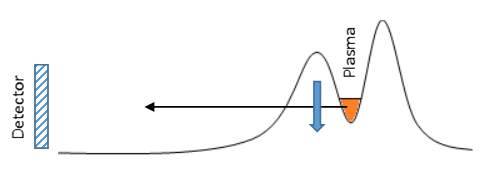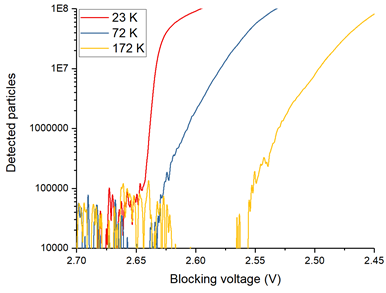# Diagnostic Tools

## Temperature measurements

The plasma temperature is an important property in antihydrogen experiments because we must achieve very low temperatures of positrons and antiprotons in order to effectively synthesize antihydrogen. The plasma temperature is related to the kinetic energy of the particles in the plasma. For a single particle, we can simply define the kinetic energy of the particle to be one half multiplied by the mass of the particle and multiplied by the square of the velocity of the particle. However, a typical plasma in our trap consists of millions of particles that have a range of kinetic energies rather than a single kinetic energy. In other words, some particles will be moving slowly and have a small kinetic energy whereas other particles will be moving very fast and have a large kinetic energy.

In order to deal with such a large range of kinetic energies we define the temperature to be a measure of the spread of kinetic energies. A high temperature plasma has a larger spread of kinetic energies than a low temperature plasma meaning that high temperature plasmas have more particles with large kinetic energies than cold plasmas have. Additionally, we can describe the temperature as a measure of the average kinetic energy of the particles in the plasma. We can diagnose the temperature of plasmas within our Penning-Malmberg trap by slowly lowering one side of the potential that confines them (the black curve in Fig 1) and measuring the rate at which particles escape. Since we know what voltage each particle escapes at, we are essentially measuring the energy distribution of the plasma. We can convert between energy and temperature using the Boltzmann constant.[Fig 1] A plasma (orange) is slowly released by lowering one side of the confining potential (black line). A detector (such as a multi-channel plate) counts the particles which escape.

Since a cold plasma has a narrow spread of particle kinetic energies, the particles in a cold plasma will escape over a narrow range of confining potentials. A warm plasma on the other hand, has a larger spread of particle kinetic energies and therefore particles in a warm plasma will escape over a much larger range of confining potentials than the cold plasma. By measuring the gradient of the escape profile (shown in Fig 2) and performing a bit of math, we can calculate the temperature of the plasma.[Fig 2] The escape profile of three different plasmas is shown as a function of the blocking voltage at which they escape (the height of the leftmost peak of Fig 1). Red = 23 K, Blue = 72 K, Yellow = 172 K.